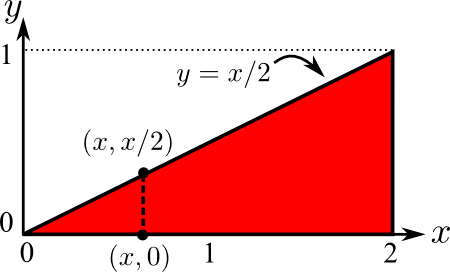# Math Insight

### Image: Double integral over triangular region, integrating x firstThe red region is a triangle over which we can compute a double integral. Since the domain is described as $0 \le x \le 2$, $0 \le y \le x/2$, it is set up to integrate with respect to $x$ first.

Image file: double_integral_over_triangle_x_first.png

Source image file: double_integral_over_triangle_x_first.svg
Source image type: Inkscape SVG